# Interconnection of Two Port Network

## Interconnection of Two Port Network:

Various types of Interconnection of Two Port Network such as series connection of two ports, parallel connection of two ports, cascade connection of two ports etc.

### Series Connection of Two Ports:

Consider two networks N’ and N” are connected in series as shown in Fig. 6.8 (a). When two ports are connected in series, we can add their z parameters to get overall z-parameter of the overall series connection.

Let the z-parameters of network N’ be z’11, z’12, z’21, z’22. Let the z parameters of network N” be z”11, z”12, z”21, z”22. Let the overall z-parameters of series connection be z11, z12, z21, z22.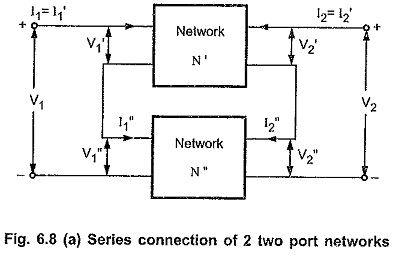For series connection we have,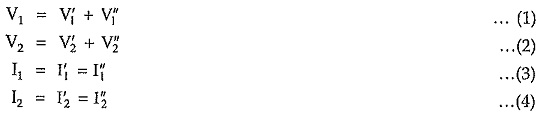For network N’ , z-parameter equations are,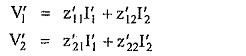For network N”, z-parameter equations are,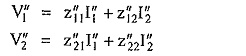From equations (1) , (2) and (3) , (4) we can write,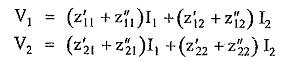In the Matrix form, above equations can be written as,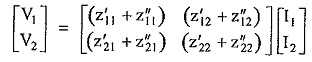Thus, overall z-parameters are,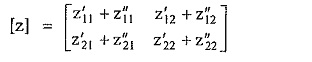Hence, the z-parameters of the series connection are the sum of z-parameters of the individual network connected in series.

### Parallel Connection of Two Ports:

Consider two networks N’ and N” are connected in parallel as shown in Fig. 6.8 (b). When two ports are connected in parallel, we can add their y-parameters to get overall y-parameters of the parallel connection.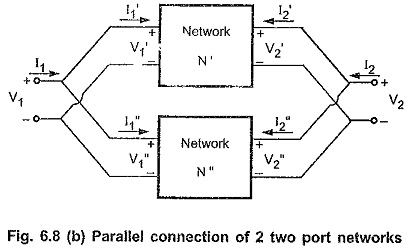Let the y-parameters of the network N’ by y’11, y’12, y’21, y’22. Let the y-parameters of the network N” be y”11, y”12, y”21, y”22. Let the overall y-parameters of parallel connection be y11, y12, y21, y22.

For parallel connection we have,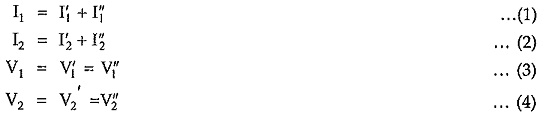For network N’, the y-parameter equations are,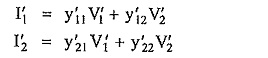For network N” the y-parameter equations are,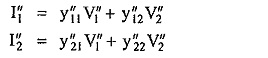From equations (1), (2) and (3), (4), we can write,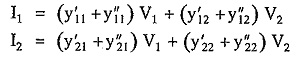In matrix form, above equations can be written as,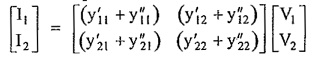Thus, overall y-parameters are,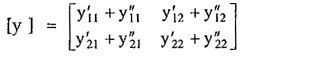Hence, the y-parameters of the parallel connection are the sum of y-parameters of the individual networks connected in parallel.

### Cascade Connection of Two Ports:

The cascade connection is also called Tandem connection. Consider two networks N’ and N” are connected in cascade as shown in Fig. 6.8 (c). When two ports are connected in cascade, we can multiply their individual transmission parameters to get overall transmission parameters of the cascade connection.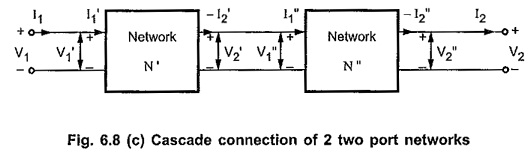Let the transmission parameters of network N’ be A’ , B’ ,C’ , D’. Let the transmission parameters of network N” be A”, B”, C”, D”. Let the overall transmission parameters of cascade connection be A, B, C, D.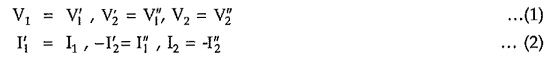For the network N’, transmission parameter equations are,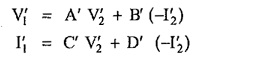For the network N”, transmission parameter equations are,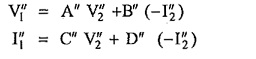The overall transmission parameters of the cascade connection can be written as,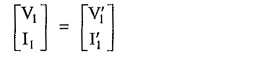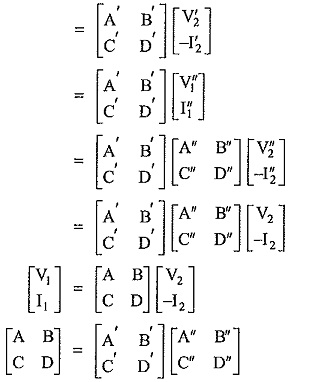Hence, the transmission parameters for the cascaded two port network is simply the matrix product of the transmission parameter matrix of each individual two port network in cascade.

### Series and Parallel Permissibility of Connection:

Before interconnecting two ports it is very important to check for the overall port if the current entering one terminal is same as that leaving other terminal of same port. This test is called as Validy test or Brune’s Test.

If the 2-port networks to be interconnected do not satisfy Brune’s test, an ideal transformer of 1:1 turns ratio is connected at one of the ports. This transformer provides necessary isolation and it also ensures that the characteristics of any network does not alter due to the interconnection with other.

The permissibility of connection of two port network through the transformer is as shown in the Fig. 6.9 (a) to (d).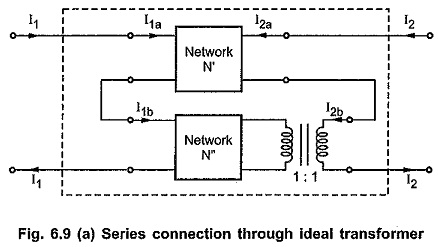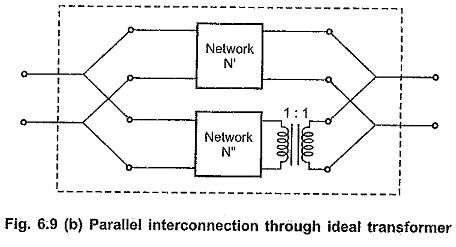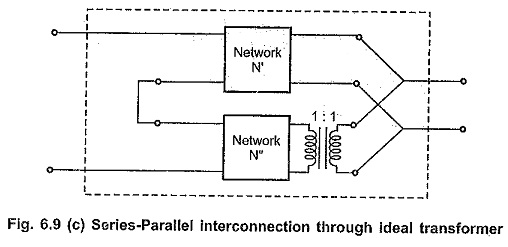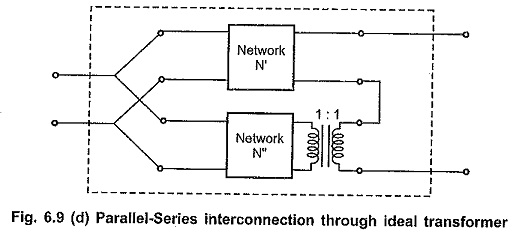Updated: November 5, 2019 — 11:46 pm